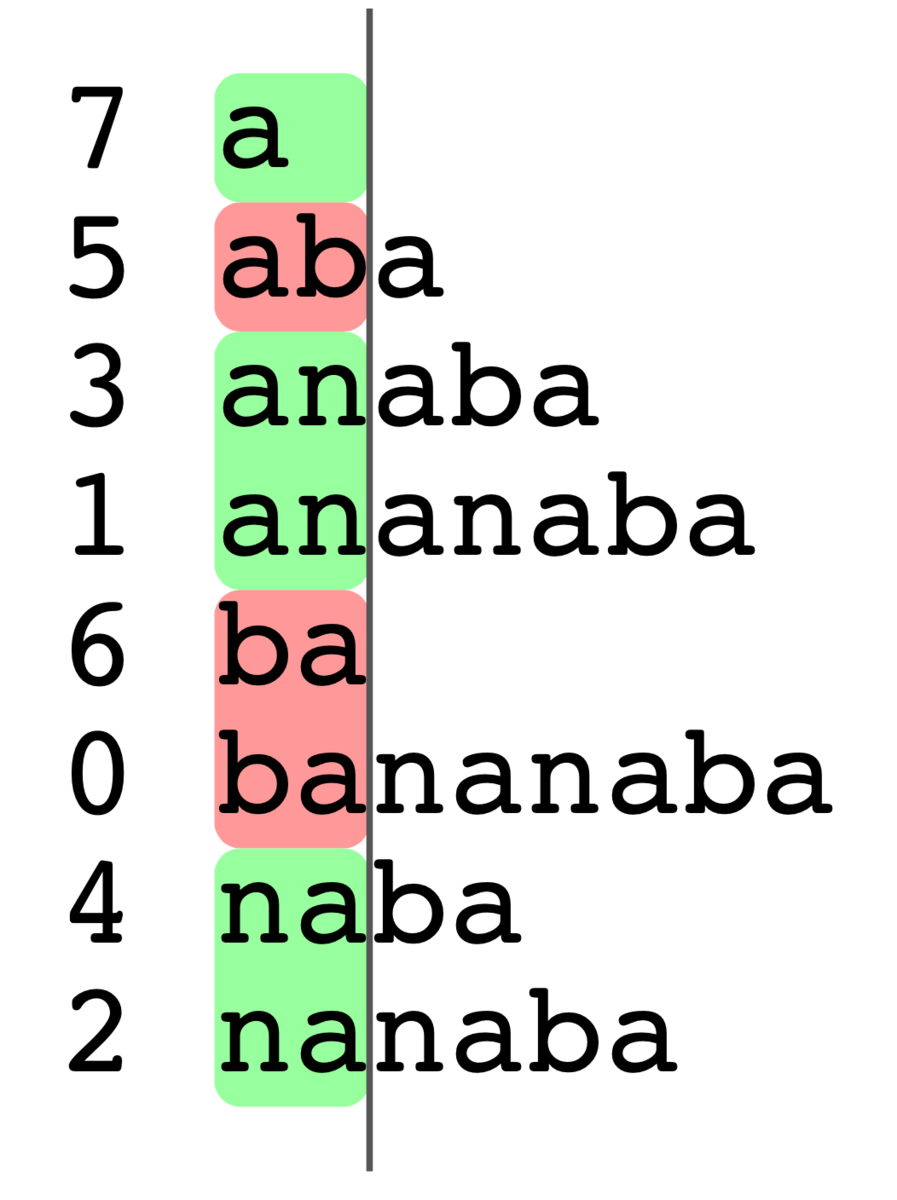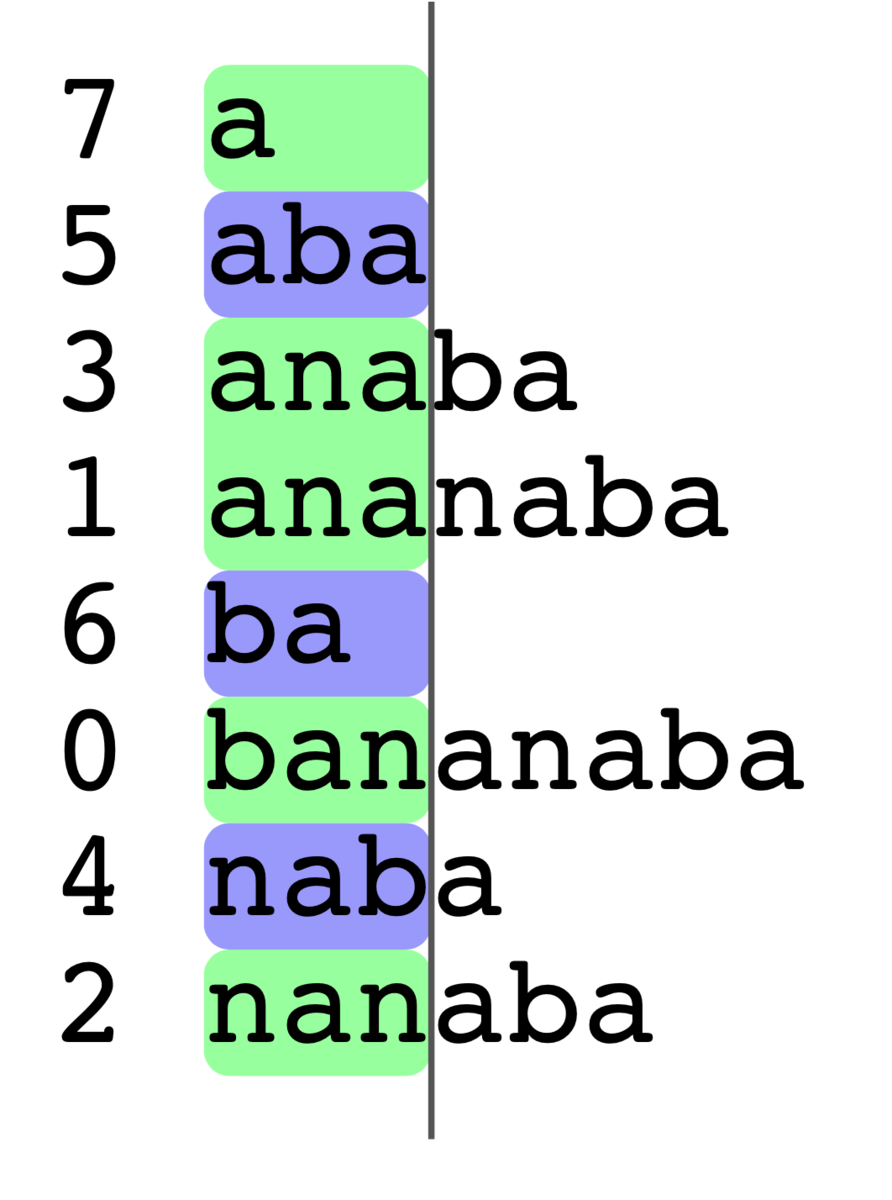# ABC141 E. Who Says a Pun?

There exists the way to find LCS (longest common prefix) using DP. LCS, LCP and edit distance are typical problems of string DP. Since the ant book describes only LCS, LCS may be not well known.

#include <bits/stdc++.h>

#define rep(i, n) for (int i = 0; i < (n); i++)
#define repr(i, n) for (int i = (n) - 1; i >= 0; i--)
#define range(a) a.begin(), a.end()

using namespace std;
using ll = long long;

int main() {
cin.tie(nullptr);
ios::sync_with_stdio(false);
int N; cin >> N;
string S; cin >> S;
vector<vector<int>> lcp(N + 1, vector<int>(N + 1));
int ans = 0;
repr(i, N) {
repr(j, N) {
if (S[i] != S[j]) {
lcp[i][j] = 0;
} else {
lcp[i][j] = lcp[i + 1][j + 1] + 1;
}
if (i < j) {
ans = max(ans, min(j - i, lcp[i][j]));
}
}
}
cout << ans << endl;
}


We can find a lot of binary search solutions, but it is unnecessarily. We can solve this problem in O(N).

For example, if you have "bananaba", its suffix array is as follows.Assume that the answer is greater or equal to 2. The pair of (i, j) must be included in the same group (see above figure.) Look at the group which contains "anana" and "ananaba". "anana" is a suffix at 3 and "ananaba" is a suffix at 1. Since abs(3-1)>=2, this assumption is correct, which concludes the answer is greater or equal to 2.

Assume that the answer is greater or equal to 3. In this case, the suffixes are decomposed intoIf the answer is 3, only ("anana", "ananaba") is possible. However, this pair doesn't give the answer because abs(3-1)<3. Therefore, this assumption is incorrect, which concludes the answer is less than 3.

#include <bits/stdc++.h>

#define rep(i, n) for (int i = 0; i < (n); i++)
#define repr(i, n) for (int i = (n) - 1; i >= 0; i--)
#define range(a) a.begin(), a.end()

using namespace std;
using ll = long long;

int main() {
cin.tie(nullptr);
ios::sync_with_stdio(false);
int N; cin >> N;
string S; cin >> S;
// SA-IS O(n)
vector<int> sa(N + 1); rep(i, N + 1) sa[i] = i;
sort(range(sa), [&](int i, int j) {
return S.substr(i) < S.substr(j);
});
// LCP O(n)
vector<int> lcp(N + 1);
rep(i, N) {
int h = 0;
while (sa[i] + h < N && sa[i + 1] + h < N && S[sa[i] + h] == S[sa[i + 1] + h]) h++;
lcp[i] = h;
}

vector<int> ord(N);
rep(i, N) ord[i] = i;
// counting sort O(n)
sort(range(ord), [&](int i, int j) { return lcp[i] > lcp[j]; });

vector<int> l(N + 1);
vector<int> r(N + 1);
vector<int> mn(N + 1);
vector<int> mx(N + 1);
rep(i, N + 1) {
l[i] = i;
r[i] = i;
mn[i] = sa[i];
mx[i] = sa[i];
}

// O(n)
int ans = 0;
for (int i : ord) {
int j = i + 1;
int k = l[i];
r[l[i]] = r[j];
l[r[j]] = l[i];
mn[k] = min(mn[k], mn[j]);
mx[k] = max(mx[k], mx[j]);
ans = max(ans, min(lcp[i], mx[k] - mn[k]));
}
cout << ans << endl;
}